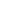Times Tables Flashcards

Category Math Flashcards

Times Tables Flashcards from 1 to 12 in Australian format and European, US and Asian countries format.

Multiplication Times Tables Posters

Category Math Posters

Times Tables Posters from Numbers 1 to 12 in Australian format and US, European and Asian Formats

Long Division Worksheets (with and without Remainders)

Worksheets of long division (with and without Remainders)

Mixed Division Worksheets (with and without Remainders)

Worksheets of mixed division (with and without Remainders)

Single Digit Quotient Simple Division Worksheets (with Remainders)

Worksheets of single digit quotient division (with Remainders)

Single Digit Quotient Simple Division Worksheets (No Remainders)

Worksheets of single digit quotient division (with no Remainders)

Visual Division Worksheets (With Remainders)

Worksheets of visual division (with Remainders)

Visual Division Worksheets (No Remainders)

Worksheets of visual division (no Remainders)

Double Digit Multiplication Worksheets

Worksheets of double digit multiplication

Single Digit Simple Multiplication Worksheets

Worksheets of single digit multiplication